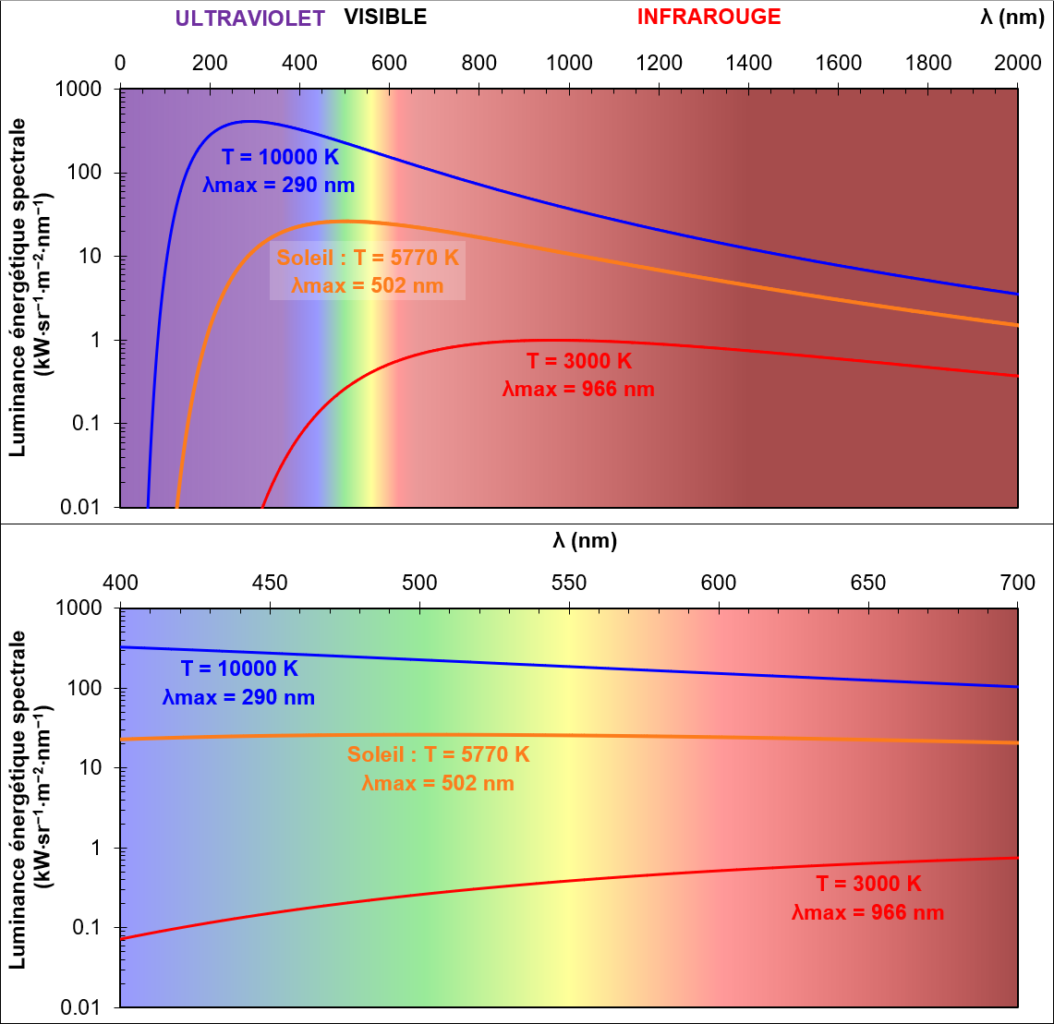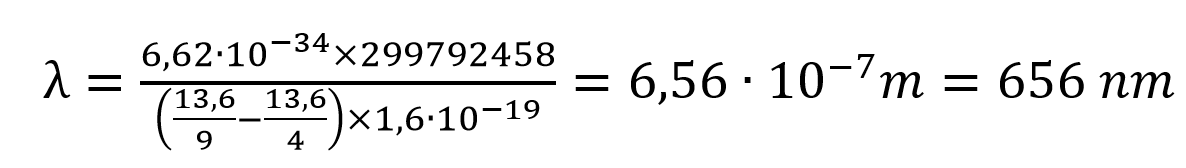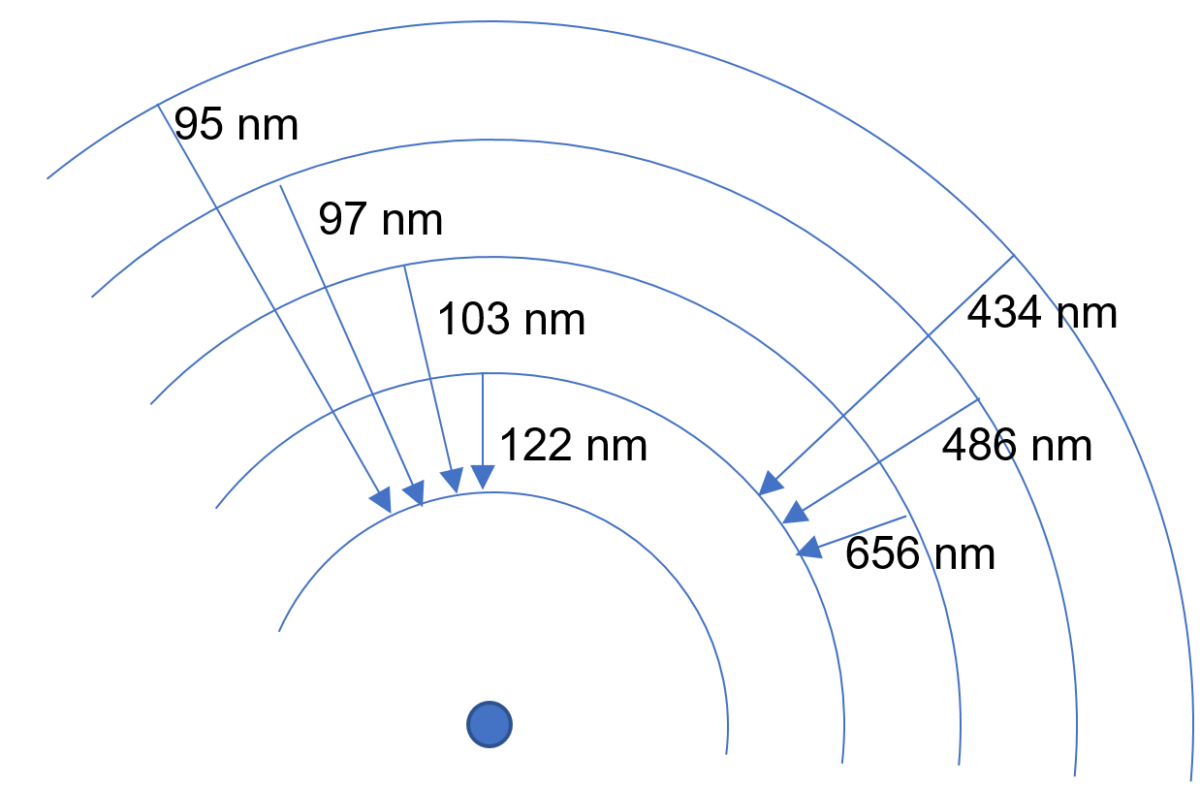# Introduction

The photon is the elementary vector particle of light. There is a direct relationship between the energy of a photon and its color (or, its wavelength, physicists will say). A blue photon carries more energy than a red photon. The more energetic a photon, the weaker (or shorter) its wavelength (represented by the Greek letter λ (lambda).

# Energy and color

The relationship between energy and wavelength is: E = h · c / λ with

• h = Planck constant (6.62 × 10-34 J s)
• c = speed of light in vacuum (299 792 458 m / s).
• λ = wavelength

A spectrum is a representation of an object’s emission level as a function of its wavelength. The wavelength range of visible photons is from 400 nm (nanometers) to 700 nm. The wavelength ranges of the different types of electromagnetic radiation (= “lights”) classified by increasing energy are:

1. Radio waves: λ> 10 m
2. Radar waves: 10 m> λ> 1 m
3. Microwave: 1 m> λ> 1 mm
4. Infrared (IR): 1 mm> λ> 700 nm
5. Visible: 700 nm> λ> 400 nm
6. Ultraviolet (UV): 400 nm> λ> 10 nm
7. X-rays: 10 nm> λ> 0.01 nm
8. Γ rays (Gamma): λ <0.01 nm

# Heat color

The two main emission processes in astronomy are:

• The black body type emission (thermal emission)
• Fluorescence

The emission of a black body is a thermal emission: any body brought to a certain temperature emits a continuum of light, which means that this emission emits photons over a wide wavelength range (or spectral range). Thus, an object heated to 20-25 ° C will emit in the infrared, around 10 µm (= 10,000 nm). That’s why we use infrared cameras when we want to see humans at night. If one takes, at random, a body brought to about 5500 ° C (or 5773 K, the Kelvin is the unit of measure of the temperature preferred by physicists: it is like the degrees Celsius to which we add 273.15), the spectrum will present a maximum emission around 500 nm, right in the middle of the visible range. 5600-5800 K is precisely the surface temperature of the sun which, roughly, emits like a black body. A hotter star will emit mainly in the UV, a colder star in the IR.# Fluorescence color

The process of fluorescence light emission is of a totally different nature since it is due to the emission of a photon by an electron which passes from an energy level of an atom or a molecule to an energy level inferior. An atom is made up of a positively charged nucleus around which “negatively charged electrons” orbit. But unlike a satellite whose orbit can be stabilized at any altitude (if there is no atmosphere to slow it down and bring it down), “altitudes” (in fact, levels of energy) to which the electrons are “allowed” to move are quantified: it is level 1 or level 2 or level 3… etc but not 1.26 or 2.73. Level 1, the lowest in energy, is called the fundamental level, the higher levels are called excited levels. And if an electron “falls” from one level to a lower level in energy, it emits a photon whose energy is strictly equal to the energy difference of the two levels between which the transition takes place. For the simplest atom, hydrogen (1 single electron orbiting a nucleus formed by a single proton), the fundamental level (n = 1) is at -13.6 eV (electron-Volt, c ‘is the atomists’ favorite unit of energy; 1 eV = 1.6 × 10-19 J)). The energy of the other levels is given by the relation E = -13.6 / n², n being the number of the level. E1 = -13,6 eV E2 = -13,6/4 eV = -3,39 eV E3 = -13,6/9 eV = -1,51 eV … Using the relation E = h · c / λ, if we convert the eV into Joules, for the transition of an electron from level 3 to level 2, we obtain:this is the wavelength of the  Hα  line !It can be noted that an electron can also descend from an excited level to the fundamental in several stages, and therefore by emitting several lower energy photons. Thus, the intensity of a line depends on the efficiency of the excitation process (for example, some atoms or molecules are easier to excite than others), and the most likely paths of de-excitation. Therefore, the most intense rays are not necessarily due to the most abundant species. Septembre 2021 @Axel Randonnier